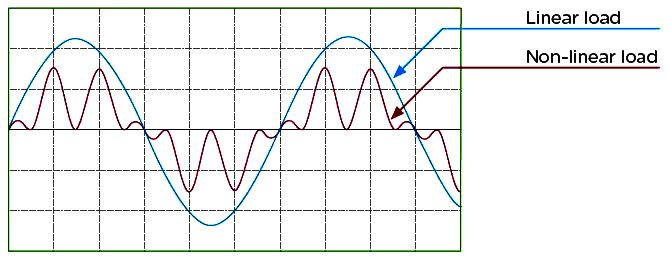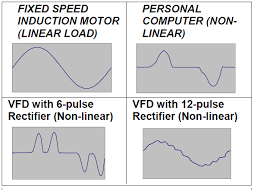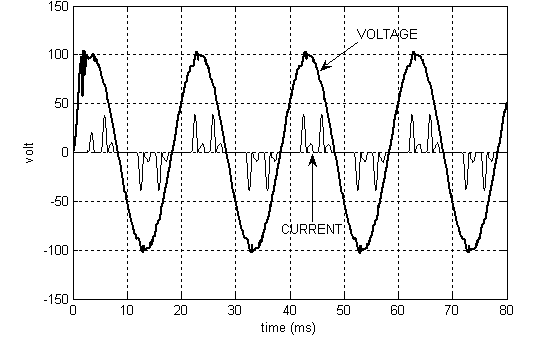# Linear and non-linear electrical loads

AC electrical loads are referred to either as linear or non-linear loads depending on how they draw current from the mains power supply waveform. Electrical products can be linear or non-linear and it is their waveforms that tell the difference between them.

These loads are both found in electrical devices in homes and businesses, but their appearance, performance, and impact on the power grid are different.A linear load is an electrical load device that, in steady-state operation, presents a complex constant load impedance to the power source throughout the cycle of applied voltage.
Linear electrical loads are defined as currents that are proportional to the voltage at any given time. This is otherwise referred to, in physics, as Ohm’s law.
In simpler words, if we give a sinusoidal voltage to a load and the load current is also sinusoidal, we call that load "linear".

Capacitor and Capacitive Circuits
Inductor and Inductive Circuits
Resistance and Resistive Circuits
Modeled Transformers
Incandescent lamps
Resistance heaters
Fans

For example, let’s say you have a waveform reflecting the voltage, with a current in clean 60 Hz sine waves. With this waveform, the current looks exactly like the voltage. Hence, the term ‘linear load’ is applied in this instance.

With these types of loads, when the applied voltage is increased, so too will the current flowing through the circuit increase. The same will apply to vice versa.

On the other spectrum, we have nonlinear electrical loads, where the current isn’t proportional to the voltage and fluctuates based upon alternating load resistance. They look nothing like the voltage on a waveform.
A nonlinear load Electrical load that draws current discontinuously or whose impedance varies throughout the cycle of the input ac voltage waveform.

Computers
Printers
Rectifiers
Servers
Televisions
Computer and server
Printer
Scanner
Television
Microwave
Switched-mode power supply such as mobile chargers, laptops, etc.
Energy saving lightbulbNonlinear electrical loads work by drawing currents in brief and rapid pulses. As these pulses distort the current’s waveforms, harmonics are generated, which can lead to power problems.Non-linear loads draw in currents in abrupt short pulses. These pulses distort the current waveforms, which in turn generates harmonics that can lead to power problems affecting both the distribution system equipment and the loads connected to it.
As the current is pulled through the system, nonlinear loads have the capacity to create current, and in turn, voltage distortion.
These power problems have the real capacity to affect distribution system equipment and also any loads connected to it. You can read our post on harmonics here.

Share